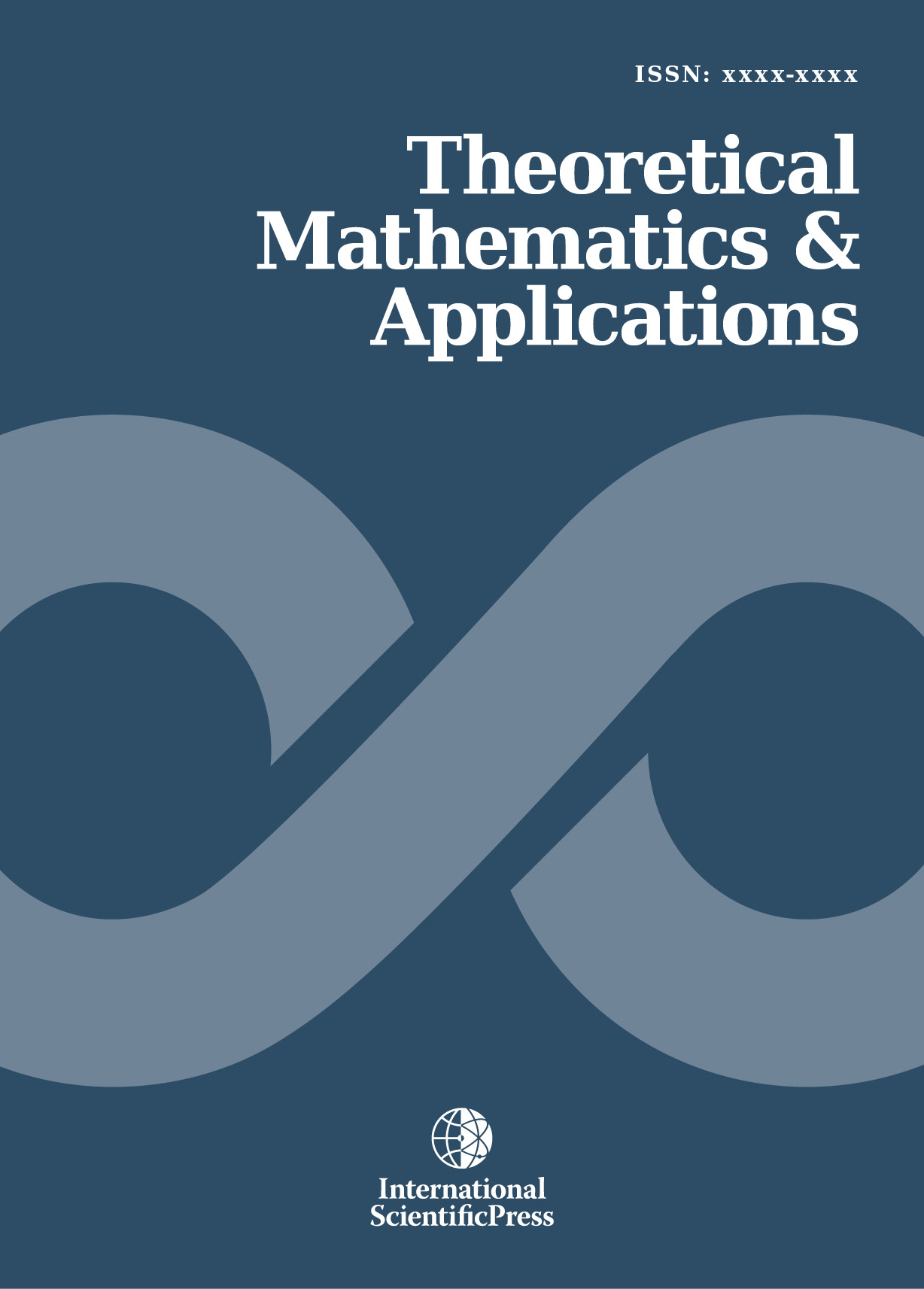# Theoretical Mathematics & Applications

#### An Algorithm for Least Squares Computation Using the Manifold and Hilbert Space Dialectics

•[ Download ]
• Times downloaded: 1817
• Abstract

The method of least squares is widely used in numerical analysis of data in all applied quantitative fields. Although there is only one least squares criterion, several schemes have been used for its implementation. The manifold approach treats the entire least squares process, including the representation of the variables, the model formation and the computations, in terms of manifolds. A manifold is a group of variables or functions taken together and treated as an entity in the computation process. This paper presents the least squares optimization on the manifolds and shows that the express formation and solution of the usually formidable normal equations can be avoided by employing the Hilbert space axioms and methods in the Euclidean space generated by the axial manifolds. The sequential and systematic approach of the new scheme, the preservation of the group structure and the analytical insights it provides for understanding the fundamental geometry of the least squares problem, all of which are demonstrated in the sample applications presented, support the conclusions that the manifold approach is less daunting, requires less core storage space and facilitate better understanding of the problem and the solution.ISSN: 1792-9687 (Print)
1792-9709 (Online)